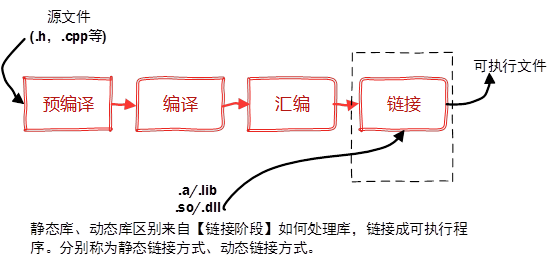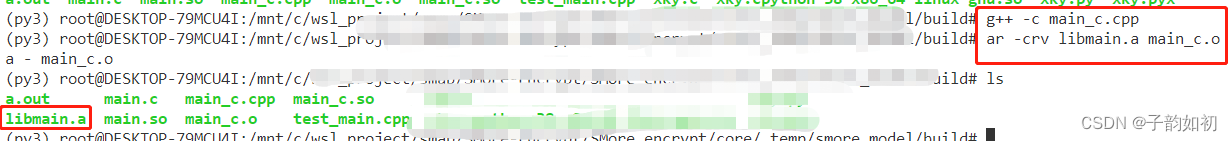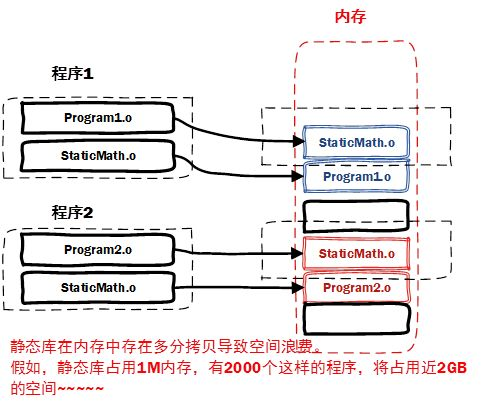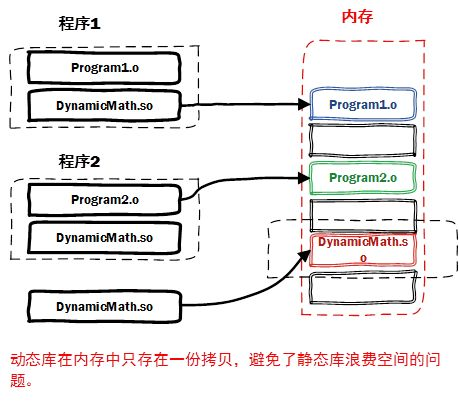# 什么是库## 静态库

•  静态库对函数库的链接是放在编译时期完成的。
• 程序在运行时与函数库再无关系，移植方便。
• 浪费空间和资源，因为所有相关的目标文件与牵涉到的函数库被链接合成一个可执行文件。
• Linux静态库命名规范，必须是"lib[your_library_name].a"：lib为前缀，中间是静态库名，扩展名为.a。

创建静态库（.a）

通过上面的流程可以知道，Linux创建静态库过程如下：

• 首先，将代码文件编译成目标文件.o（StaticMath.o）
•         g++ -c main_c.cpp

注意带参数-c，否则直接编译为可执行文件

• 通过ar工具将目标文件打包成.a静态库文件
•         ar -crv libmain_c.a main_c.o

生成静态库libmain_c.a## 动态库另一个问题是静态库对程序的更新、部署和发布页会带来麻烦。如果静态库liba.lib更新了，所以使用它的应用程序都需要重新编译、发布给用户（对于玩家来说，可能是一个很小的改动，却导致整个程序重新下载，全量更新）。

动态库在程序编译时并不会被连接到目标代码中，而是在程序运行是才被载入。不同的应用程序如果调用相同的库，那么在内存里只需要有一份该共享库的实例，规避了空间浪费问题。动态库在程序运行是才被载入，也解决了静态库对程序的更新、部署和发布页会带来麻烦。用户只需要更新动态库即可，增量更新动态库特点

• 动态库把对一些库函数的链接载入推迟到程序运行的时期
• 可以实现进程之间的资源共享。共享库
• 程序升级变得简单
• 链接载入完全由程序员在程序代码中控制（显式调用
• ## C++中创建动态库

如果是cpp还要加上extern "C"，因此C编译器编译后在符号库中的名字为_foo，而C++编译器为了实现重载则会产生像_foo_int_int之类的名称，因此如果使用g++或者cpp编译动态链接库，则会找不到对应函数出现Segmentation fault

同时要注意编译器的选择，虽然都不会报错，但是执行逻辑不一样

• g++ 会把 .c 文件当做是 C++ 语言 (在 .c 文件前后分别加上 -xc++ 和 -xnone, 强行变成 C++), 从而调用 cc1plus 进行编译
• 遇到 .cpp 文件也会当做是 C++, 调用 cc1plus 进行编译
• 还会默认告诉链接器, 让它链接上 C++ 标准库
• gcc 会把 .c 文件当做是 C 语言. 从而调用 cc1 进行编译
• gcc 遇到 .cpp 文件, 会处理成 C++ 语言. 调用 cc1plus 进行编译
• gcc 默认不会链接上 C++ 标准库
• 编写gen.c

``````int gen(){
return 1;
}``````

编译.c为.so文件

``gcc  gen.c -o gen.so -g -Wall -fPIC -shared``

编写test_main.cpp文件

``````#include <dlfcn.h>

#include <iostream>

typedef int (*PYMAIN)();
using namespace std;
int main() {
PYMAIN py_main = NULL;
void* handle = dlopen("./gen.so", RTLD_LAZY);
if (!handle) {
std::cout << dlerror();
};
py_main = (PYMAIN)dlsym(handle, "gen");
std::cout << py_main << std::endl;
int r = py_main();
std::cout << "load dll : " << r << std::endl;
return 0;
}``````

编译cpp文件

``g++ test_main.cpp -ldl -g   && ./a.out``

# Cython配合Python-C接口加载动态链接

1.  编写gen.pyx文件或gen.py文件，注意这里不能用cpdef或者cdef，因为python runtime会找不到

``````def gen():
return 5``````

2. 通过cython转换为.c后编译为动态链接库test_gen.cpython-38-x86_64-linux-gnu.so

``````cython -3 gen.py
g++ gen.c -g -I/usr/include/python3.8 -lpython3.8   -Wall -fPIC -shared ``````

如果需要编译project需则需要设置Extension module

``````modules = [Extension(
os.path.splitext(file_name).replace(os.sep, "."),
[input_path])]
#mod: utils.ops ['numpy_demo/utils/ops.py']

cythonize(modules,language='c++',language_level=3)``````

3. 编写test_main.cpp代码

``````#include <Python.h>
#include <dlfcn.h>
#include <numpy/arrayobject.h>

using namespace std;
void print_dict(PyObject* obj) {
if (!PyDict_Check(obj)) return;
PyObject *k, *keys;
keys = PyDict_Keys(obj);
for (int i = 0; i < PyList_GET_SIZE(keys); i++) {
k = PyList_GET_ITEM(keys, i);
const char* c_name = _PyUnicode_AsString(k);
printf("%s\n", c_name);
}
}
int main() {
Py_Initialize();
//   PyRun_SimpleString("import sys");
//   PyRun_SimpleString("sys.path.append('./')");
PyObject *p_name, *p_module, *p_dict, *p_func, *p_args, *p_res, *numpy_array ;
p_module = PyImport_Import(p_name);
p_dict = PyModule_GetDict(p_module);
print_dict(pDict);
p_func = PyDict_GetItemString(p_dict, "gen");
p_res= PyObject_CallObject(p_func, p_args);
numpy_array = PyArray_FROM_OTF(p_res, NPY_DOUBLE, 0);
int dim = PyArray_NDIM(p_res);
printf("%ld", dim);
Py_Finalize();
return 0;
}``````

4. 设置python环境编译运行

``````export PYTHONPATH=./
g++ test_main.cpp -ldl -g -I/usr/include/python3.8 -lpython3.8 && ./a.out``````

来源：子韵如初